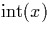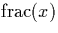Next: Signed binary integers to Up: Conversion to and from Previous: Decimal fractions to signed

## Signed Binary to Decimal

In order to convert signed binary numerals into decimal we proceed as follows. First of all we separate the integer and fractional parts of the signed binary input using the exponent of the (mantissa, exponent) representation. The integer and fractional part are handled separately. The integer part of the signed binary representation is finite, and can be converted into decimal relatively easily. In order to convert the fractional part we first convert it from a signed binary stream into a signed decimal stream. Then we take a finite portion of this and change the signed decimal into decimal. Lastly we combine the integer and fractional part, handling and overflow from the fractional to the integer part, and generate the complete decimal output.

The algorithms used here are modifications of those given by Knuth  for converting between bases using unsigned digit representations.

We use the notationto denote the operation which integer part of a real x, andthe operation which returns its fractional part.

Martin Escardo
5/11/2000